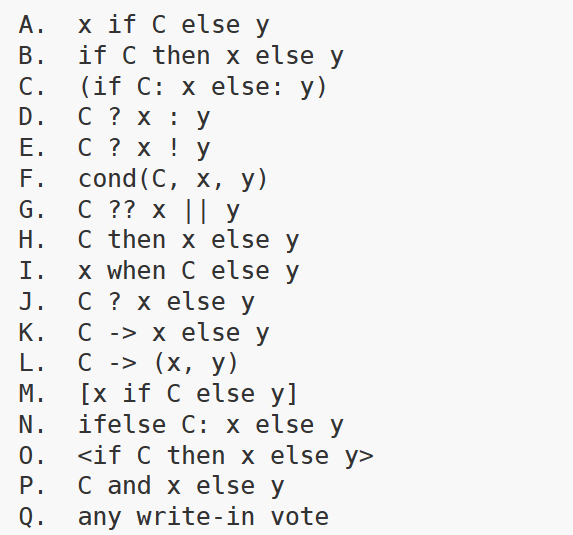• 为什么 Python 不支持三元运算符呢？
• 发布于 1个月前
• 80 热度
0 评论

```// 常规 if-else
if (a > b) {
result = x;
} else {
result = y;
}

// 简化后的写法
result = a > b ? x : y;```

Python 社区的投票
Python 发布于 1991 年，但在接下来的 15 年里，除了 if-else 语法外，它并不支持三元运算符和其它条件表达式。而且，在 2006 年引入条件表达式前，社区对此进行了漫长而曲折的争论，可以说这是一个设计得很艰难的语法了。

（1）使用标点符号构建的三元运算符

`<condition> ? <expression1> : <expression2>`

（2）使用现有和新的关键字构建

`<condition> then <expression1> else <expression2>`

（3）其它思路

```(if <condition>: <expression1> else: <expression2>)
<condition> and <expression1> else <expression2>
<expression1> if <condition> else <expression2>
cond(<condition>, <expression1>, <expression2>)```and-or 用于条件选择的问题

```a = True and True or "Python猫"
b = True and False or "Python猫"```

X if C else Y 非常易于理解，可读性高。它延续了“明确优于隐式”的风格，使用了直观口语化的“if-else”，而不是引入可能引起混淆的标点符号，就像 Python 选择“and”和“or”两个单词，而不是“&&”和“||”两个符号，它们有着异曲同工之妙。

```my_str = ""
# 中间存在其它代码逻辑
# 当 condition 为真时，变量会被重新赋值
my_str = "Python猫" if condition```

Go、Rust 为什么不支持三元运算符？

Go 语言不支持“?:”运算符，而是推荐使用原生的“if-else”写法。文档的解释很简短，只有一段话：Go 语言没有 ?: 运算符，因为语言的设计者们经常看到它被用来创建难以理解的复杂表达式。虽然 if-else 形式比较长，但是它无疑更清晰易懂。一个语言只需要一个条件控制流结构。

```// 若条件为真，得到 5，否则 6
let number = if condition { 5 } else { 6 };```

```let x = 42;
let result = if x > 50 {
println!("x is greater than 50");
x * 2 // 这是一个表达式，将返回的值赋给 result
} else {
println!("x is less than or equal to 50");
x / 2 // 也是一个表达式，将返回的值赋给 result
};```